## Newton Raphson Method for Load Flow Analysis:

The Newton Raphson Method for Load Flow Analysis is a powerful method of solving non-linear algebraic equations. It works faster and is sure to converge in most cases as compared to the GS method. It is indeed the practical method of load flow solution of large power networks. Its only drawback is the large requirement of computer memory which has been overcome through a compact storage scheme. Convergence can be considerably speeded up by performing the first iteration through the GS method and using the values so obtained for starting the NR iterations. Before explaining how the NR method is applied to solve the load flow problem, it is useful to review the method in its general form.

Consider a set of n non-linear algebraic equations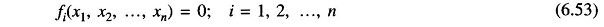Assume initial values of unknowns as x01, x02, …,x0n.

Let Δx01, Δx02, …, Δx0n be the corrections, which on being added to the initial guess, give the actual solution. Therefore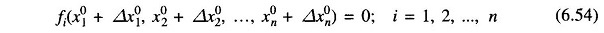Expanding these equations in Taylor series around the initial guess, we have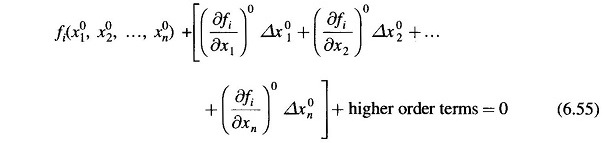whereare the derivatives of fi with respect to x1, x2, …, xn evaluated at (x01, x02, …, x0n).

Neglecting higher order terms we can write Eq. (6.55) in matrix form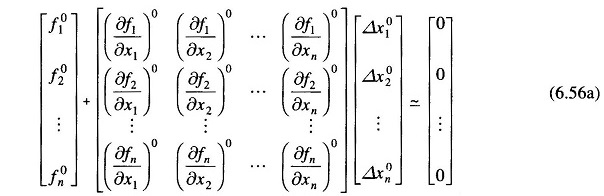or in vector matrix form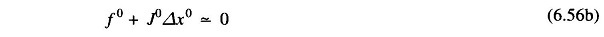J0 is known as the Jacobian matrix (obtained by differentiating the function vector f with respect to x and evaluating it at x0). Equation (6.56b) can be written as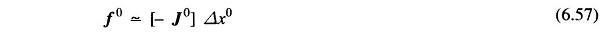Approximate values of corrections Δx0 can be obtained from Eq (6.57). These being a set of linear algebraic equations can be solved efficiently by triangularization and back substitution.

Updated values of x are then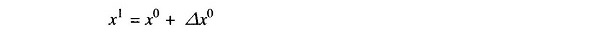or, in general, for the (r + 1)th iteration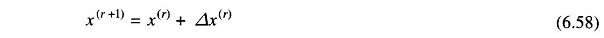Iterations are continued till Eq. (6.53) is satisfied to any desired accuracy, i.e.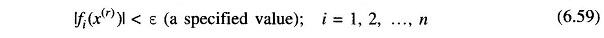### NR Algorithm for Load flow Solution:

First, assume that all buses are PQ buses. At any PQ bus the load flow solution must satisfy the following non-linear algebraic equations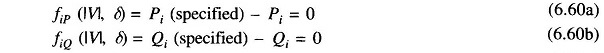where expressions for Pi and Qi are given in Eqs. (6.27) and (6.28). For a trial  set of variables |Vi|, δi, the vector of residuals f0 of Eq. (6.57) corresponds to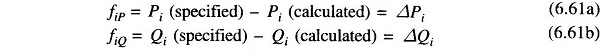while the vector of corrections , Δx0 corresponds to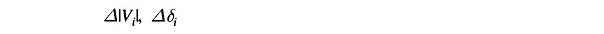Equation (6.57) for obtaining the approximate corrections vector can be written for the load flow case as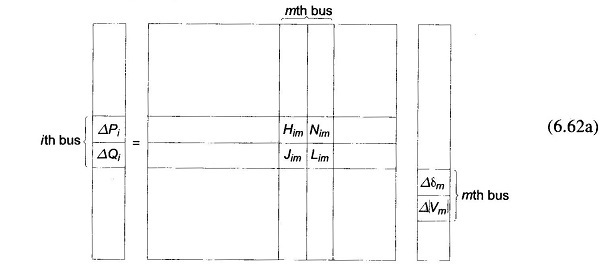where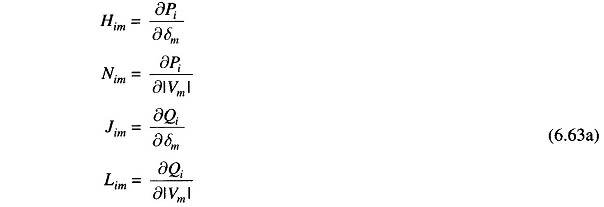It is to be immediately observed that the Jacobian elements corresponding to the ith bus residuals and mth bus corrections are a 2 x 2 matrix enclosed in the box in Eq. (6.62a) where i and m are both PQ buses.

Since at the slack bus (bus number 1), P1 and Q1 are unspecified and |V1|, δ1 are fixed, there are no equations corresponding to Eq. (6.60) at this bus. Hence the slack bus does not enter the Jacobian in Eq. (6.62a).

Consider Newton Raphson Method for Load Flow Analysis Formula now the presence of PV buses. If the ith bus is a PV bus, Qi is unspecified so that there is no equation corresponding to Eq. (6.60b) for this bus. Therefore, the Jacobian elements of the ith bus become a single row pertaining to ΔPi , i.e.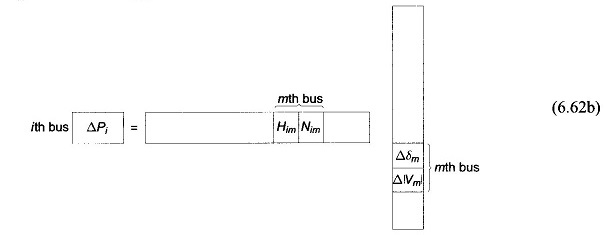If the mth bus is also a PV bus, |Vm| becomes fixed so that Δ|Vm| = 0. We can now write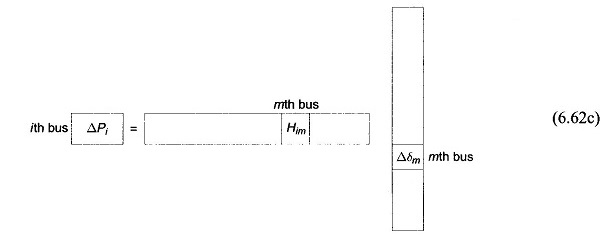Also if the ith bus is a PQ bus while the mth bus is a PV bus, we can then write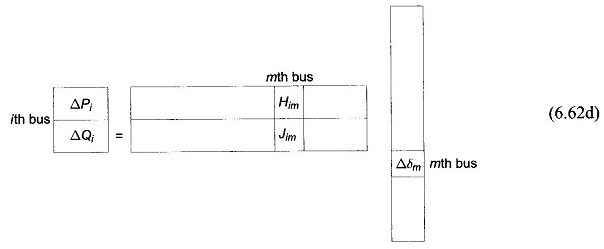It is convenient for numerical solution to normalize the voltage corrections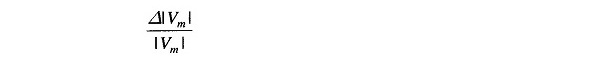as a consequence of which, the corresponding Jacobian elements become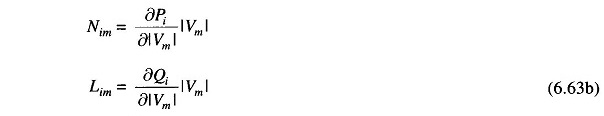Expressions for elements of the Jacobian (in normalized form) of the load flow Eqs. (6.60a and b) are given below:

Case 1:where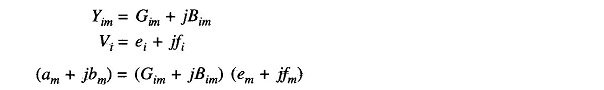Case 2: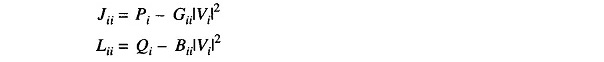An important observation can be made in respect of the Jacobian by examination of the YBUS matrix. If buses i and m are not connected, Yim = 0 (Gim = Bim = 0). Hence from Eqs. (6.63) and (6.64), we can write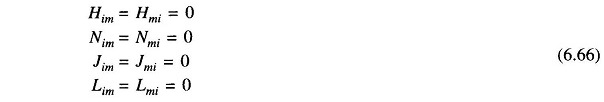Thus the Jacobian is as sparse as the YBUS matrix.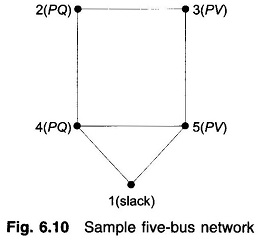Formation of Eq. (6.62) of the NR method is best illustrated by a problem. Figure 6.10 shows a five-bus power network with bus types indicated therein. The matrix equation for determining the vector of corrections from the vector of residuals is given below.

Corresponding to a particular vector of variables [δ2|V23δ4|V45]T, the vector of residuals [ΔP2 ΔQ2 ΔP3 ΔP4 ΔQ4 ΔP5]T and the Jacobian (6 x 6 in this example) are computed. Equation (6.67) is then solved by triangularization and back substitution procedure to obtain the vector of corrections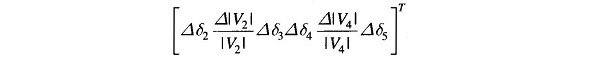Corrections are then added to update the vector of variables.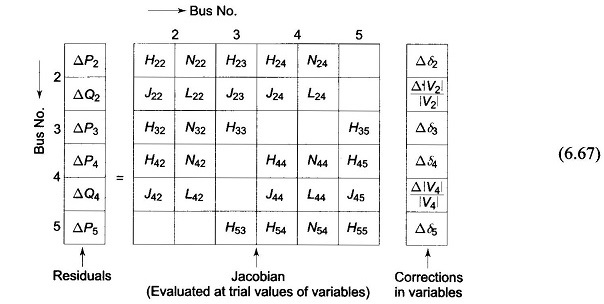### Iterative Algorithm:

Omitting programming details, the iterative algorithm for the solution of the load flow problem by the NR method is as follows:

1. With voltage and angle (usually δ = 0) at slack bus fixed, assume |V|, δ at all PQ buses and δ at all PV In the absence of any other information flat voltage start is recommended.
2. Compute ΔPi (for PV and PQ buses) and ΔQi, (for all PQ buses) from (6.60a and b). If all the values are less than the prescribed tolerance, stop the iterations, calculate P1 and Q1 and print the entire solution including line flows.
3. If the convergence criterion is not satisfied, evaluate elements of the Jacobian using Eqs. (6.64) and (6.65).
4. Solve Eq. (6.67) for corrections of voltage angles and magnitudes.
5. Update voltage angles and magnitudes by adding the corresponding changes to the previous values and return to step 2.
Scroll to Top